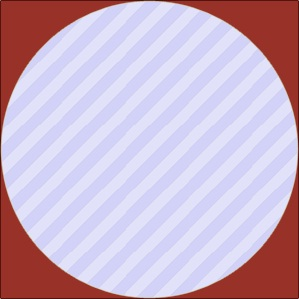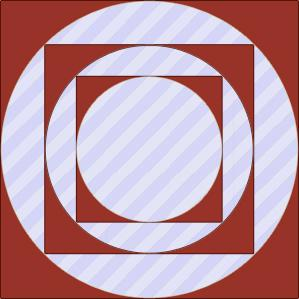# 程序设计能力实训

1116. 圆和正方形$K = 3$ 时，图形为：### 输入格式

$1$ 行：整数 $T$ ($1 \le T \le 10$) 为问题数

$2$ ~ $T+1$ 行：每行包括两个整数 $N$$K$，分别对应上面描述中的边长和操作数 $(N\le 10000,K\le 100000)$

### 样例

Input
3
10 1
10 2
10 3

Output
case #0:
21.460184
case #1:
32.190275
case #2:
37.555321